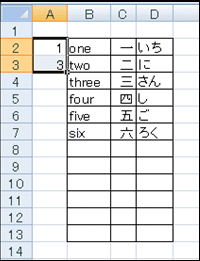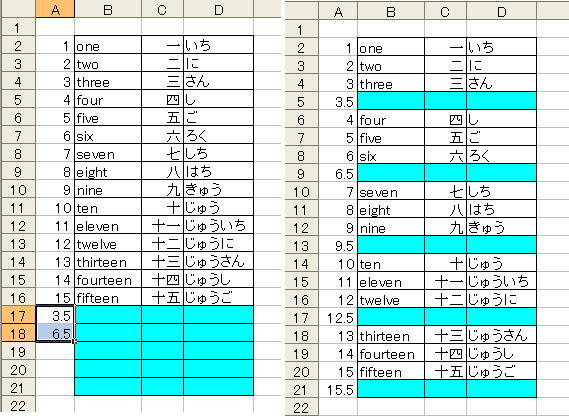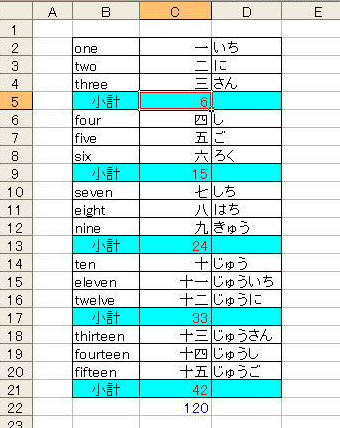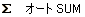Japanese

## Add Rows at fixed interval and Subtotal

An insertion of rows at constant intervals is irritating if there are so many lines. An ascending sort technique is proposed here for row insertion.
RELATED PAFGE　Row deletion at fixed interval

### Row Insertion at every other line

Figure 1.As demonstrated in 4th frame of Figure 1, Cells A2 〜 A7 are filled with odd numbers, whereas cells A8 〜 A13 are with even numbers.

Select entire Table and an ascending order of "A Column" is chosen for sorting.

Meaningless contents of "A Column" are preferable to delete after completion.

### Case of every fourth line

Figure 2.Similar to the previous section, at an "A Column" a simple permutation is filled up to the bottom of the table.

At A17 and A18, type 3.5 and 6.5.

These cells are copied up to the proper cell using Auto Fill Technique.

Contents of A17 and a18 cells are needless to say available using figures as [ 3.1 and 6.1 ] or otherwise.

The subsequent procedure is as similar as that of the previous section.

A drawback of this method is as follow.
Each inserted line lacks a ruled line unless border lines are drawn previously

Case of previous section (every other line), an use of [ 1.5, 2.5 ] at A17 and A18 cells in Figure 2 is also available.

Case of every third line, use [ 2.5, 4.5 ] sequences at A17 and A18 cells in Figure 2.

Case of every fifth line, [ 4.5, 8.5 ] and sixth, [ 5.5, 10.5 ] and so on.

If the above mentioned method is performed continuously, it is also possible to insert sequential vacant lines.

### Insertion of Subtotal

Figure 3.As demonstrated in Figure 3, the use of(Auto SUM) command or typing 『 =subtotal(9, c2:c4) 』 at C5 cell gives rise to an appearance of "6". Afterwards copy this content to C9, C13, C17, C21 cells.

In C22 cell, 『 =SUM(C21,C17,C13,C9,C5) 』 is automatically entered if you use(Auto SUM) command.